# Calculating Aggregate Control Times¶

In some simulations and assignments, control junctions are not explicitly considered, either because it is not possible to evaluate a control junction or because it is not meaningful for the project. For example, this is the case with a static assignment where queues cannot be modeled and therefore no specific time can be used to evaluate the control plan.

To overcome this, you can use time-aggregated control quantities instead of time-specific ones. In particular, consider calculating the following aggregates:

• Average green time (seconds)
• Average min. green time (seconds)
• Average max. green time (seconds)
• Average yellow duration (seconds)
• Average cycle duration (seconds)
• Control junction type
• Uncontrolled time (seconds)

## Average calculation¶

Let T be the duration of the whole period. Let Vi be a quantity associated with the i-th subperiod (phase) where it is active, and let di be the duration of this period. The averages are calculated by explicitly applying the following formula: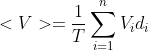where the durations di play the role of weights of the average. In the case of a multi-ring control junction, the final average will be the average of the average of each ring.

We have two ways of finding this average, depending on how detailed you need the calculation to be: an approximate method and an exact method. The exact method produces more accurate results for intervals containing fractions of cycles.

#### Approximate method¶

In this method, Vi is considered to be the cycle average of V.

This method is simpler and faster but less accurate. It is suitable for averages over long periods and when there are few or no changes of traffic control.

#### Exact method¶

In this method, Vi is the average over the period defined by di.

This method is more accurate but more complex and costly to calculate. It is more suitable for short periods, where such a degree of accuracy is useful, and when there might be changes to control plans or other modifications to traffic control in the intersection.

### Examples¶

This calculation can be very complicated, depending on the different control plans in use. As an example, consider a situation where you want to calculate the average green time of the turn shown below.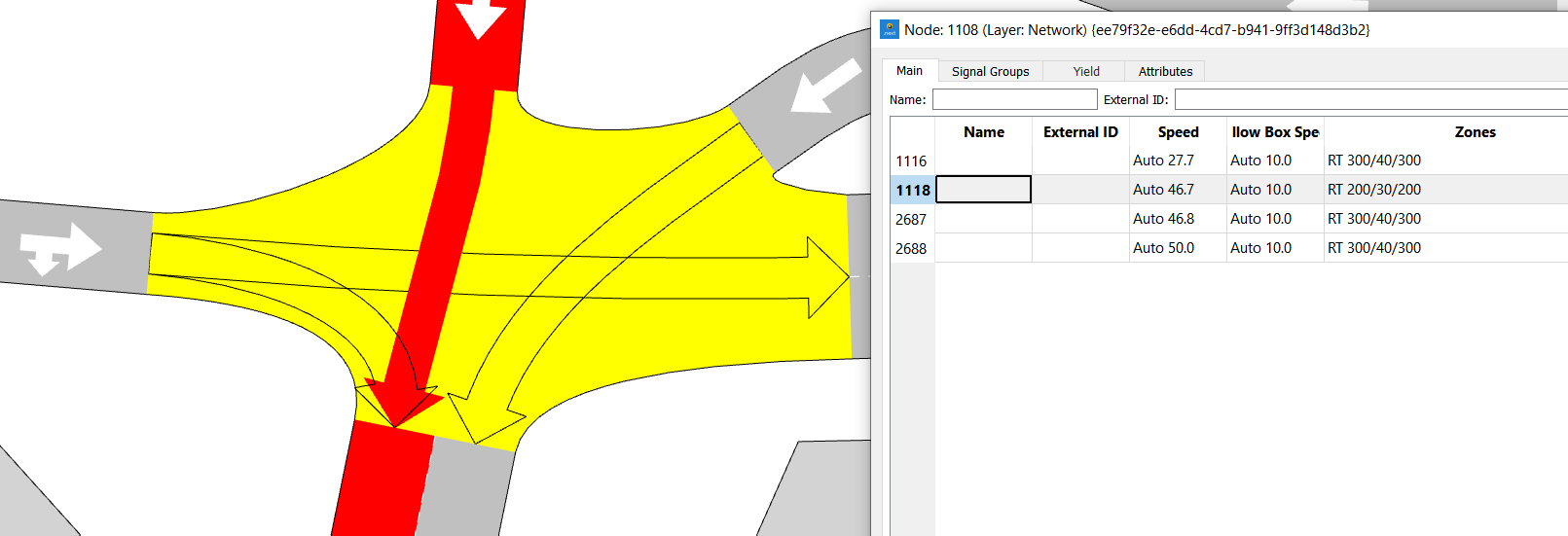The control is defined in the master control plan below.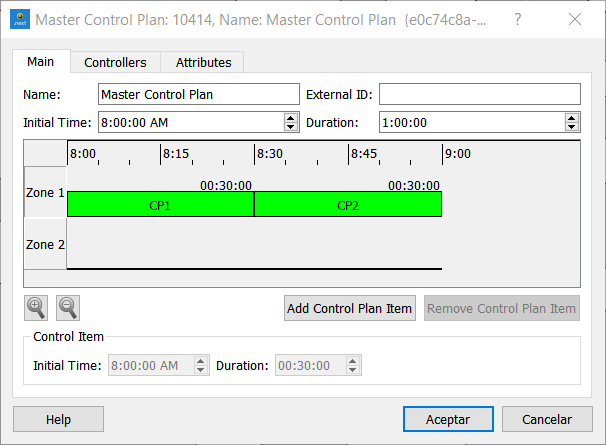The control junction in CP1 is as follows.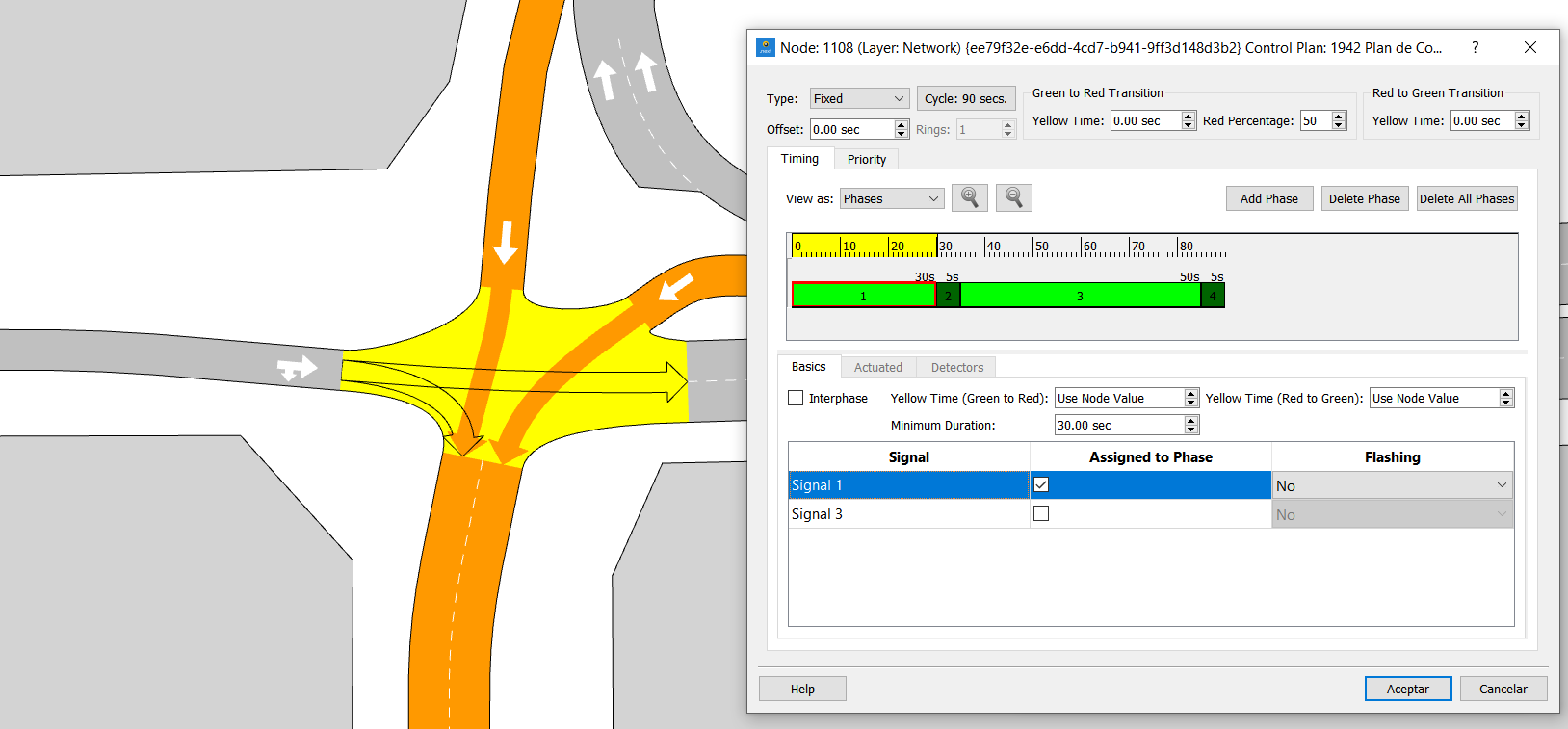And in CP2 as below.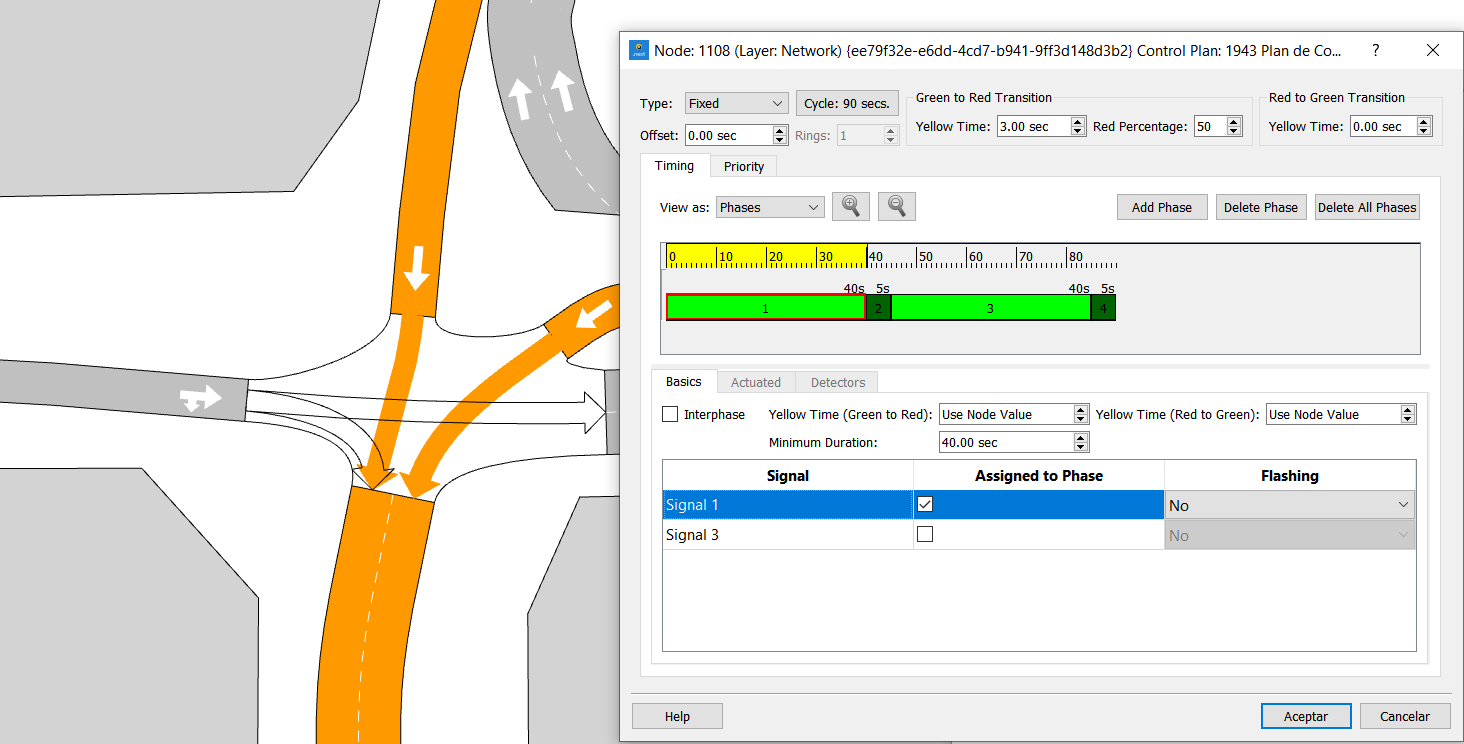If you want to know the average green time of this turn over the whole hour (3,600s), where the MCP is defined, apply the previous formula with these considerations:

• For CP1, 20 cycles of 90 seconds pass. Each contributes 30 seconds of green time for this turn.

• For CP2, 20 cycles of 90 seconds pass. Each contributes 40 seconds of green time for this turn.

When calculating the average, the result for both the approximate and the exact method is shown below.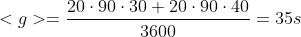That was a simple case. Now consider a case where the average green time is between 08:20 and 08:50 (1,800s). In this case, the approximate and exact methods will not give the same values.

Approximate method:

• For CP1, six full cycles of 90 seconds and 2/3 of a cycle (60 seconds) pass. Each full cycle contributes 30 seconds of green time for this turn, with the partial cycle contributing proportionally.

• For CP2, 13 full cycles of 90 seconds and 1/3 of a cycle (30 seconds) pass. Each full cycle contributes 40 seconds of green time for this turn, with the partial cycle contributing proportionally.

When calculating the average, the result is shown below.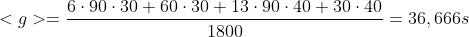Exact method:

• The starting cycle time for CP1 is mod(20min·60s/min,90s)=60s and the ending cycle time is mod(30min·60s/min,90s)=0s. Therefore, we have a contribution of [60s, 90s] (0s of green) and 6 full cycles (30s of green).

• The starting cycle time for CP2 is mod(30min·60s/min,90s)=0s and the ending cycle time is mod(50min·60s/min,90s)=60s. Therefore, we have a contribution of 13 full cycles (30s of green) and [0s, 60s] (30s of green).

When calculating the average, the result is shown below.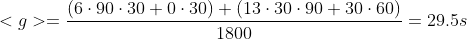Comparing both results, it is clear that in this case the approximate method overestimates the average green time for the turn.

## Control Junction Type Calculation¶

In a time period there might be more than one control junction defined (at different times) for a given node. In general we cannot associate a single junction type with a node. Instead, if all types of control junction during the period are equal, we consider the control junction to belong to this type. If this is not the case, the control-junction type is undetermined (-1).

## Uncontrolled Time Calculation¶

In a time period there might be time intervals where no control is defined for a given node. The sum of the duration of all such intervals adds up to the total uncontrolled time. This quantity might be useful when considering how relevant the previously calculated averages are, among others.